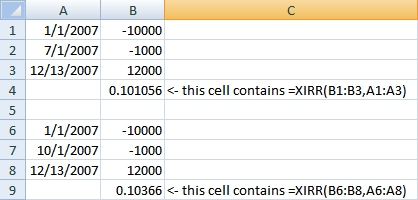# Calculate Rate of Return When You’ve Added or Removed Money

At the beginning of each year, I like to calculate the rate of return of each of my accounts for the previous year. If I haven’t added to or subtracted from an account, that’s as easy as (gain or loss) divided by initial investment. So, if I started with \$10,000 and ended up with \$12,000, my return was \$2000 / \$10000 = 0.20, or 20%.

But what if I added \$1000 at some point during the year? Depending on when I added the \$1000, it would be contributing various amounts to my return. This is where Excel’s XIRR function becomes very handy (other spreadsheets have it too). The XIRR function is used to determine the rate of return with irregular payments (IRR can be used if the payments are on a regular schedule).

I found a good explanation of calculating investment rate of return here, but really like Allen Wyatt’s ExcelTips article on the XIRR function. He explains how you use the function–really, you just need to remember that money put in the account is expressed as negative numbers, and money pulled out of the account (or remaining at the end) is a positive. You give the XIRR function the dates and amounts for the start, payments/withdrawals, and ending value, and it gives you a rate of return.

Notice in the following example how I started with \$10,000, added \$1000, and ended up with \$12,000. Depending on when I added the \$1000, the return varied from 10.1% to almost 10.4%.Allen Wyatt has WordTips and ExcelTips that are searchable. Check out www.vitalnews.com.